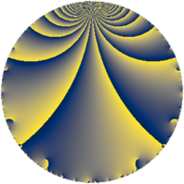# Properties

 Label 2001.2.a.eLevel $2001$ Weight $2$ Character orbit 2001.a Self dual yes Analytic conductor $15.978$ Analytic rank $1$ Dimension $2$ CM no Inner twists $1$

# Related objects

## Newspace parameters

 Level: $$N$$ $$=$$ $$2001 = 3 \cdot 23 \cdot 29$$ Weight: $$k$$ $$=$$ $$2$$ Character orbit: $$[\chi]$$ $$=$$ 2001.a (trivial)

## Newform invariants

 Self dual: yes Analytic conductor: $$15.9780654445$$ Analytic rank: $$1$$ Dimension: $$2$$ Coefficient field: $$\Q(\sqrt{6})$$ Defining polynomial: $$x^{2} - 6$$ Coefficient ring: $$\Z[a_1, \ldots, a_{5}]$$ Coefficient ring index: $$1$$ Twist minimal: yes Fricke sign: $$1$$ Sato-Tate group: $\mathrm{SU}(2)$

## $q$-expansion

Coefficients of the $$q$$-expansion are expressed in terms of $$\beta = \sqrt{6}$$. We also show the integral $$q$$-expansion of the trace form.

 $$f(q)$$ $$=$$ $$q + q^{3} -2 q^{4} -\beta q^{5} + ( -2 + \beta ) q^{7} + q^{9} +O(q^{10})$$ $$q + q^{3} -2 q^{4} -\beta q^{5} + ( -2 + \beta ) q^{7} + q^{9} -2 q^{12} + q^{13} -\beta q^{15} + 4 q^{16} + ( 1 + \beta ) q^{17} - q^{19} + 2 \beta q^{20} + ( -2 + \beta ) q^{21} - q^{23} + q^{25} + q^{27} + ( 4 - 2 \beta ) q^{28} + q^{29} + ( -8 + \beta ) q^{31} + ( -6 + 2 \beta ) q^{35} -2 q^{36} + ( 5 - 2 \beta ) q^{37} + q^{39} + ( -2 + 3 \beta ) q^{41} + ( -5 - 2 \beta ) q^{43} -\beta q^{45} + ( -10 + \beta ) q^{47} + 4 q^{48} + ( 3 - 4 \beta ) q^{49} + ( 1 + \beta ) q^{51} -2 q^{52} -2 q^{53} - q^{57} + ( -3 - \beta ) q^{59} + 2 \beta q^{60} + ( -8 - 2 \beta ) q^{61} + ( -2 + \beta ) q^{63} -8 q^{64} -\beta q^{65} + ( -4 + 2 \beta ) q^{67} + ( -2 - 2 \beta ) q^{68} - q^{69} + ( -5 + 3 \beta ) q^{71} + ( -2 + 5 \beta ) q^{73} + q^{75} + 2 q^{76} + ( 1 - 2 \beta ) q^{79} -4 \beta q^{80} + q^{81} + ( -10 - \beta ) q^{83} + ( 4 - 2 \beta ) q^{84} + ( -6 - \beta ) q^{85} + q^{87} + ( 3 - \beta ) q^{89} + ( -2 + \beta ) q^{91} + 2 q^{92} + ( -8 + \beta ) q^{93} + \beta q^{95} + ( 12 - 2 \beta ) q^{97} +O(q^{100})$$ $$\operatorname{Tr}(f)(q)$$ $$=$$ $$2q + 2q^{3} - 4q^{4} - 4q^{7} + 2q^{9} + O(q^{10})$$ $$2q + 2q^{3} - 4q^{4} - 4q^{7} + 2q^{9} - 4q^{12} + 2q^{13} + 8q^{16} + 2q^{17} - 2q^{19} - 4q^{21} - 2q^{23} + 2q^{25} + 2q^{27} + 8q^{28} + 2q^{29} - 16q^{31} - 12q^{35} - 4q^{36} + 10q^{37} + 2q^{39} - 4q^{41} - 10q^{43} - 20q^{47} + 8q^{48} + 6q^{49} + 2q^{51} - 4q^{52} - 4q^{53} - 2q^{57} - 6q^{59} - 16q^{61} - 4q^{63} - 16q^{64} - 8q^{67} - 4q^{68} - 2q^{69} - 10q^{71} - 4q^{73} + 2q^{75} + 4q^{76} + 2q^{79} + 2q^{81} - 20q^{83} + 8q^{84} - 12q^{85} + 2q^{87} + 6q^{89} - 4q^{91} + 4q^{92} - 16q^{93} + 24q^{97} + O(q^{100})$$

## Embeddings

For each embedding $$\iota_m$$ of the coefficient field, the values $$\iota_m(a_n)$$ are shown below.

For more information on an embedded modular form you can click on its label.

Label $$\iota_m(\nu)$$ $$a_{2}$$ $$a_{3}$$ $$a_{4}$$ $$a_{5}$$ $$a_{6}$$ $$a_{7}$$ $$a_{8}$$ $$a_{9}$$ $$a_{10}$$
1.1
 2.44949 −2.44949
0 1.00000 −2.00000 −2.44949 0 0.449490 0 1.00000 0
1.2 0 1.00000 −2.00000 2.44949 0 −4.44949 0 1.00000 0
 $$n$$: e.g. 2-40 or 990-1000 Significant digits: Format: Complex embeddings Normalized embeddings Satake parameters Satake angles

## Atkin-Lehner signs

$$p$$ Sign
$$3$$ $$-1$$
$$23$$ $$1$$
$$29$$ $$-1$$

## Inner twists

This newform does not admit any (nontrivial) inner twists.

## Twists

By twisting character orbit
Char Parity Ord Mult Type Twist Min Dim
1.a even 1 1 trivial 2001.2.a.e 2
3.b odd 2 1 6003.2.a.e 2

By twisted newform orbit
Twist Min Dim Char Parity Ord Mult Type
2001.2.a.e 2 1.a even 1 1 trivial
6003.2.a.e 2 3.b odd 2 1

## Hecke kernels

This newform subspace can be constructed as the intersection of the kernels of the following linear operators acting on $$S_{2}^{\mathrm{new}}(\Gamma_0(2001))$$:

 $$T_{2}$$ $$T_{5}^{2} - 6$$

## Hecke characteristic polynomials

$p$ $F_p(T)$
$2$ $$T^{2}$$
$3$ $$( -1 + T )^{2}$$
$5$ $$-6 + T^{2}$$
$7$ $$-2 + 4 T + T^{2}$$
$11$ $$T^{2}$$
$13$ $$( -1 + T )^{2}$$
$17$ $$-5 - 2 T + T^{2}$$
$19$ $$( 1 + T )^{2}$$
$23$ $$( 1 + T )^{2}$$
$29$ $$( -1 + T )^{2}$$
$31$ $$58 + 16 T + T^{2}$$
$37$ $$1 - 10 T + T^{2}$$
$41$ $$-50 + 4 T + T^{2}$$
$43$ $$1 + 10 T + T^{2}$$
$47$ $$94 + 20 T + T^{2}$$
$53$ $$( 2 + T )^{2}$$
$59$ $$3 + 6 T + T^{2}$$
$61$ $$40 + 16 T + T^{2}$$
$67$ $$-8 + 8 T + T^{2}$$
$71$ $$-29 + 10 T + T^{2}$$
$73$ $$-146 + 4 T + T^{2}$$
$79$ $$-23 - 2 T + T^{2}$$
$83$ $$94 + 20 T + T^{2}$$
$89$ $$3 - 6 T + T^{2}$$
$97$ $$120 - 24 T + T^{2}$$